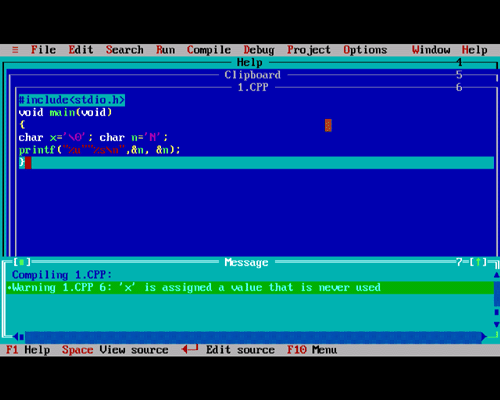# C programming Language Algorithms Multiple Choice Questions(MCQs) and Answers

Questions
1 Given in the following code segment:

```	void main(void)
{
char x='\0';
char n='N';
printf("%u""%s\n",&n, &n);
}
```

What will be the result of execution?

A ddddd N(where d represents any digit)
B 78 N
C 78 garbage
D compilation error2 Given in the following program:

```	main()
{
int x=49;
for (;x;)
x--;
printf("%d\n",x);
}
```

What will be the output of the program?

A 49
B 0
C -49
D none of these

For all non-zero values of x the for will execute. x is decremented from 49 to 0. Hence 0 is printed in the screen.

3 Given in the following program:

```	main()
{
int x=3, y=2;
dp(x/y)
}
```

What will be the output of the program?

A prints x/y=1
B prints #e=1.5
C prints #x/y=1
D none of tehes

4 In the following code segment:

```	int z,x=5,y=-10,a=4,b=2;
z=x++ - --y* b/a;
```

What will be the final value of z?

A 5
B 6
C 10
D 11

The post increment operator is associated with the variable x. So the current variable is considered i.e. x=5.

The pre increment operator is associated with the variable y. So the value of y decreses by 1. That is y=-11.

Multiplication operator and division operator has the same precedence. So the first one is executed first.

So the expression is:

z=5-(-11)*2/4

=5-(-22/4)

=5-(-5)

=10

5 Suppose that x is initialized as:

```	short int x; /*assume x is 16 bits in size*/
What is maximum number that can be printed using
printf("%d\n",x);
```
A 127
B 128
C 255
D 32767

By default short integer is signed. 1 bit is reserveed for sign i.e. only 15 bits are available.

So, the largest number will be:

215+214+..........+upto 20

i.e. 32767

### Chapters